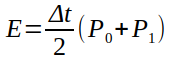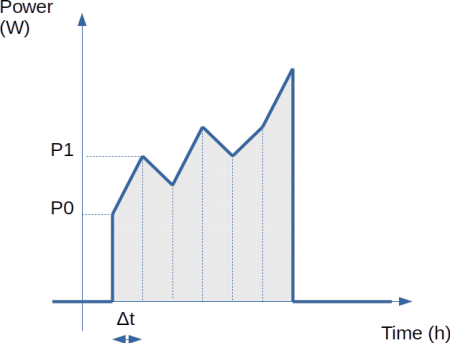# Compute Energy used using a RPICT

We will introduce how Energy can be calculated using a RPICT board. The widely known kWh - Kilo-Watt-hour. Our example will use a RPICT3T1 but this can easily apply to other models.

RPICT being a sensor interface board it does not compute energy for performances reason. We consider this sort of computation to be the duty of the recording platform and not the acquisition unit.

With Emoncms you will find this computation readily available. We refer to the Emoncms website for more details about this. This is also possible in Grafana where you can use an integral function to estimate energy from power data source.

Here we cover the computation of energy using a small Python program.

### How energy relates to power

Power is expressed in Watt (W) and energy is expressed in Watt-hour (Wh).

One single Watt-hour is obtained when a device uses 1W of power during a 1 hour period of time. The energy is graphically represented as the area below the power curve as shown below. In other words energy is the integral of power.

### Computing energy from power readings

With the RPICT board power is measured on a given time interval Δt. Every 5 seconds for example.Between 2 power readings (say P0 and P1) the energy can be calculated using the formulae below.We then use this subsequently to each data-points entry to know the energy since the beginning of the dataset. We sum up all energy calculated on each Δt to get this result.The formulae to sum up all added energies can be written like this below.### Using a python program to calculate energy

The code below will output instant power from CT1 and the accumulated energy in Wh using the formulae above.

```wget lechacal.com/RPICT/example/energy_demo.py.zip
unzip energy_demo.py.zip
```

Then run the script by calling it

```./energy_demo.py
```

Note the following

• Accumulated energy starts at zero when the program starts. If the program is restarted energy returns to zero again.
• If you prefer kWh just divide the energy by 1000 in the code.

``` #!/usr/bin/python2
import serial
ser = serial.Serial('/dev/ttyAMA0', 38400)

# Polling interval is 2 seconds
delta_T = 2./3600

# This will keep the sum of powers
P_sum = 0

# Memory for previous power reading
prev_P = 0

try:
while 1:
# Read one line from the serial buffer

# Remove the trailing carriage return line feed
line = line[:-2]

# Create an array of the data
Z = line.split(' ')

# Picking CT1 only and converting from string to float
P_CT1 = float(Z)

# Building the sum of powers
P_sum += prev_P + P_CT1

# Saving the new Power reading as previous for next loop run
prev_P = P_CT1

# Finally computing energy
E = delta_T / 2 * P_sum

# Print it nicely
print ("----------")

print ("Instant Power: %s (W)" % P_CT1)

print ("Accum Energy: %.2f (Wh)" % E)

except KeyboardInterrupt:
ser.close()```

### Calculate energy from the arduino sketch

We are demonstrating how energy can be calculated using an arduino sketch with a RPICT8 Version 3 in the link below.

### Splitting positive and negative Energy

If there is a need to distinguish between positive and negative energy from two power reading with different sign the formulae below must be used.